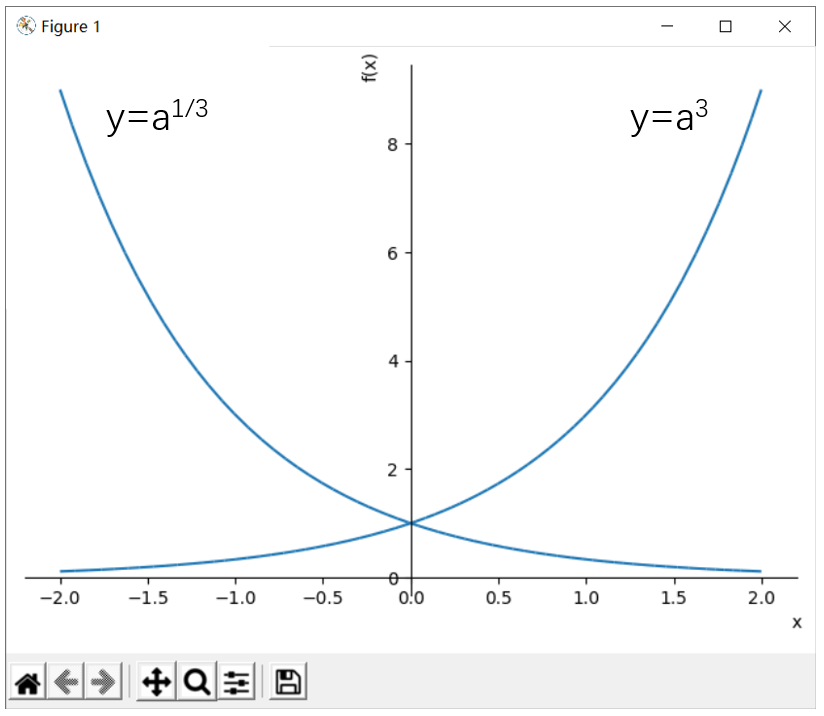978字，阅读需时4分钟y = a^x (a是常数，且a>0,a!=1)

```# 导入sympy库
from sympy import symbols,sin,plot
# 定义指数函数
def func(y,x):
return y**x
# 定义数学符号x,y
x=symbols('x')
y=symbols('y')
# 生成指数函数公式
f=func(1/3,x)
# 绘制图形
plot(f,(x,-2,2))``````# 生成指数函数公式
f1=func(1/3,x)
f2=func(3,x)
# 绘制图形
plot(f1,f2,(x,-2,2))```y=（1/a）^x = a^-x，所以y=a^x的图像与y=(1/a)^x的图像关于Y轴对称。也可以说底互为倒数的两个函数图像关于Y轴对称。

```import sympy
# 生成指数函数公式
f=func(sympy.E,x)
# 绘制图形
plot(f,(x,-2,2))```

E是sympy预定义的自然常数e，使用E需要导入sympy库。